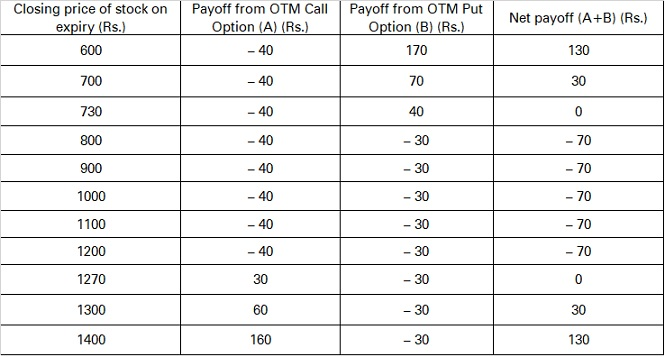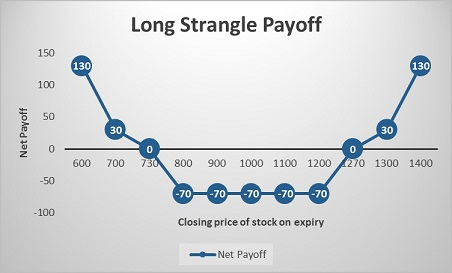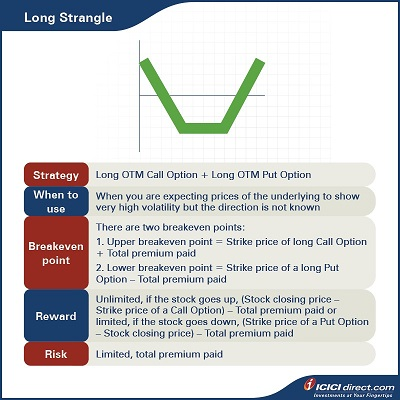This is an Options strategy for a scenario in which you are expecting significant volatility in the underlying asset but are unsure of your view of the market. Here, we will explore one such multi-leg Options strategy for this scenario - A Long Strangle.

## Long Strangle

Strangle is a strategy that is similar to Straddle but is slightly less expensive. The Options that a trader buys, or sells are normally OTM that are cheaper than ATM Options in case of a Straddle.

The trader will use Long Strangle by buying OTM Call and Put Options with the view that the prices of the underlying will show very high volatility, but the direction is unknown.

• The risk of a Long Strangle is limited to the extent of premium paid, and the rewards are unlimited. A Short Strangle, on the other hand, can experience an unlimited loss with rewards capped up to the premium received.

Strategy: Long OTM Call Option (Leg 1) + Long OTM Put Option (Leg 2)

When to use: When you are expecting the prices of the underlying to show very high volatility, but the direction is not known

Breakeven: There are two breakeven points:

1. Upper breakeven point = Strike price of long Call Option + Total premium paid

2. Lower breakeven point = Strike price of a long Put Option – Total premium paid

Maximum profit: Unlimited, if the stock goes up, (Stock closing price – Strike price of a Call Option) – Total premium paid or limited, if the stock goes down, (Strike price of a Put Option – Stock closing price) – Total premium paid

Maximum risk: Limited, total premium paid

### Let’s understand a Long Strangle strategy with an example:

Assume that the spot price of ABC Ltd. is Rs. 1,000. You buy an ABC Ltd. OTM Call of strike price Rs. 1,200 at Rs. 40 and Put Option of strike price Rs. 800 at Rs. 30. You pay a total premium of Rs. 40 + Rs. 30 = Rs. 70, and this will be the maximum loss. You will earn a profit if the stock moves more than 70 points in any direction i.e., either above Rs. 1,270 or below Rs. 730.

### Let’s look at the cash flow in various scenarios:If the stock closes at Rs. 700 on expiry: Leg 1 expires OTM while leg 2 expires ITM Let’s understand the payoff in various scenarios. It will give you a fair idea of how we have arrived at the above values.

Leg 1: Premium paid on the OTM Call Option of strike price Rs. 1200 = Rs. 40

Premium received on the OTM Call Option of strike price Rs. 1200 at expiry = Max {0, (Spot price – Strike price)} = Max {0, (700 – 1200)} = Max (0, – 500) = 0

So, the payoff from the OTM Call Option = Premium received – Premium paid = 0 – 40 = – Rs. 40

Leg 2: Premium paid on the OTM Put Option of strike price Rs. 800 = Rs. 30

Premium received on OTM Put Option of strike price Rs. 800 at expiry = Max {0, (Strike price – Spot price)} = Max {0, (800 – 700)} = Max (0, 100) = Rs. 100

So, the payoff from the OTM Put Option = Premium received – Premium paid = 100 – 30 = Rs. 70

Net Payoff = Payoff from the OTM Call Option + Payoff from the OTM Put Option = (– 40) + 70 = Rs. 30

### If the stock closes at Rs. 900 on expiry: Both the legs expire OTM

Leg 1: Premium paid on the OTM Call Option of strike price Rs. 1200 = Rs. 40

Premium received on OTM Call Option of strike price Rs. 1200 at expiry = Max {0, (Spot price – Strike price)} = Max {0, (900 – 1200)} = Max (0, – 300) = 0

So, the payoff from the OTM Call Option = Premium received – Premium paid = 0 – 40 = – Rs. 40

Leg 2: Premium paid on the OTM Put Option of strike price Rs. 800 = Rs. 30

Premium received on OTM Put Option of strike price Rs. 800 at expiry = Max {0, (Strike price – Spot price)} = Max {0, (800 – 900)} = Max (0, – 100) = 0

So, the payoff from the OTM Put Option = Premium received – Premium paid = 0 – 30 = – Rs. 30

Net payoff = Payoff from OTM Call Option + Payoff from OTM Put Option = (– 40) + (– 30) = – Rs. 70

### If the stock closes at Rs. 1300 on expiry: Leg 1 expires ITM while leg 2 expires OTM

Leg 1: Premium paid on the OTM Call Option of strike price Rs. 1200 = Rs. 40

Premium received on OTM Call Option of strike price Rs. 1200 at expiry = Max {0, (Spot price – Strike price)} = Max {0, (1300 – 1200)} = Max (0, 100) = Rs. 100

So, the payoff from the OTM Call Option = Premium received – Premium paid = 100 – 40 = Rs. 60

Leg 2: Premium paid on the OTM Put Option of strike price Rs. 800 = Rs. 30

Premium received on OTM Put Option of strike price Rs. 800 at expiry = Max {0, (Strike price – Spot price)} = Max {0, (800 – 1300)} = Max (0, – 500) = 0

So, the payoff from the OTM Put Option = Premium received – Premium paid = 0 – 30 = – Rs. 30

Net payoff = Payoff from OTM Call Option + Payoff from OTM Put Option = 60 + (– 30) = Rs. 30## Summary• Strangle is a strategy that is similar to a straddle but is slightly less expensive. The Options that a trader buys or sells are normally OTM that are cheaper than ATM Options in case of a straddle.
• The trader will use the Long Strangle by buying OTM Call and Put Options with the view that the prices of the underlying will show very high volatility, but the direction is not known.
• Breakeven: There are two breakeven points:
• Upper breakeven point = Strike price of long Call Option + Total premium paid
• Lower breakeven point = Strike price of a long Put Option – Total premium paid
• Maximum profit: Unlimited, (Stock closing price – Strike price of a Call Option) – Total premium paid or (Strike price of a Put Option – Stock closing price) – Total premium paid
• Maximum risk: Limited, total premium paid

There is one more multi-leg Option strategy for a view similar to Long Straddles and Long Strangles. It’s called the Short Call Butterfly. In the next chapter will explore this strategy.

Disclaimer:

ICICI Securities Ltd. ( I-Sec). Registered office of I-Sec is at ICICI Securities Ltd. - ICICI Venture House, Appasaheb Marathe Marg, Prabhadevi, Mumbai - 400 025, India, Tel No : 022 - 2288 2460, 022 - 2288 2470. The contents herein above shall not be considered as an invitation or persuasion to trade or invest.  I-Sec and affiliates accept no liabilities for any loss or damage of any kind arising out of any actions taken in reliance thereon. The contents herein above are solely for informational purpose and may not be used or considered as an offer document or solicitation of offer to buy or sell or subscribe for securities or other financial instruments or any other product. Investments in securities market are subject to market risks, read all the related documents carefully before investing. The contents herein mentioned are solely for informational and educational purpose.